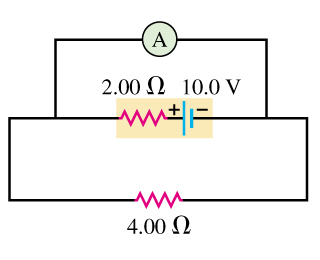# Problem: An idealized ammeter is connected to a battery as shown in the figure (Figure 1).Part A. Find the reading of the ammeter.Part B. Find the current through the 4.00-Ω resistor.Part C. Find the terminal voltage of the battery.

###### FREE Expert Solution

Current through the ammeter:

$\overline{){\mathbf{i}}{\mathbf{=}}\frac{\mathbf{\epsilon }}{\mathbf{r}}}$

Terminal voltage:

$\overline{){\mathbf{V}}{\mathbf{=}}{\mathbf{\epsilon }}{\mathbf{-}}{\mathbf{i}}{\mathbf{r}}}$

Part A

For an ideal ammeter, the resistance is zero.

100% (147 ratings)###### Problem Details

An idealized ammeter is connected to a battery as shown in the figure (Figure 1).Part A. Find the reading of the ammeter.

Part B. Find the current through the 4.00-Ω resistor.

Part C. Find the terminal voltage of the battery.